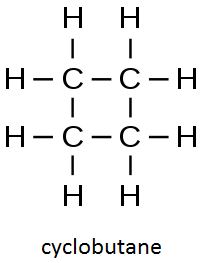# How would you draw constitutional isomers for the molecular formula C4H8?

Dec 4, 2015

There are five constitutional isomers with the formula ${\text{C"_4"H}}_{8}$.

#### Explanation:

An alkane with 4 carbon atoms would have the formula ${\text{C"_4"H}}_{10}$.

This hydrocarbon has two hydrogens less, so it must contain either a double bond or a ring.

The possibilities are:

But-1-ene

${\text{CH"_3"CH"_2"CH=CH}}_{2}$

But-2-ene

${\text{CH"_3"CH=CHCH}}_{3}$

2-MethylpropeneCyclobutaneMethylcyclopropane